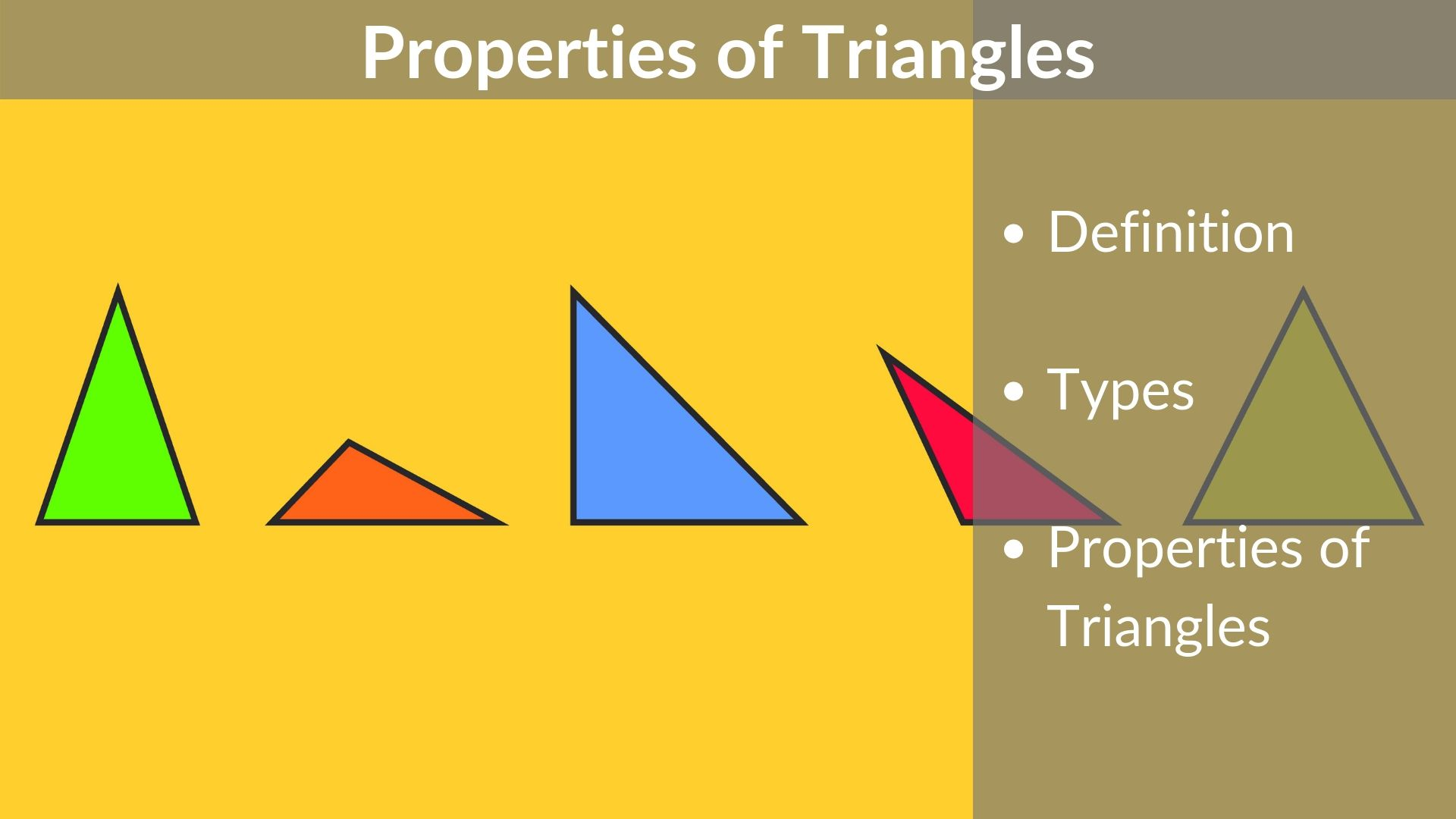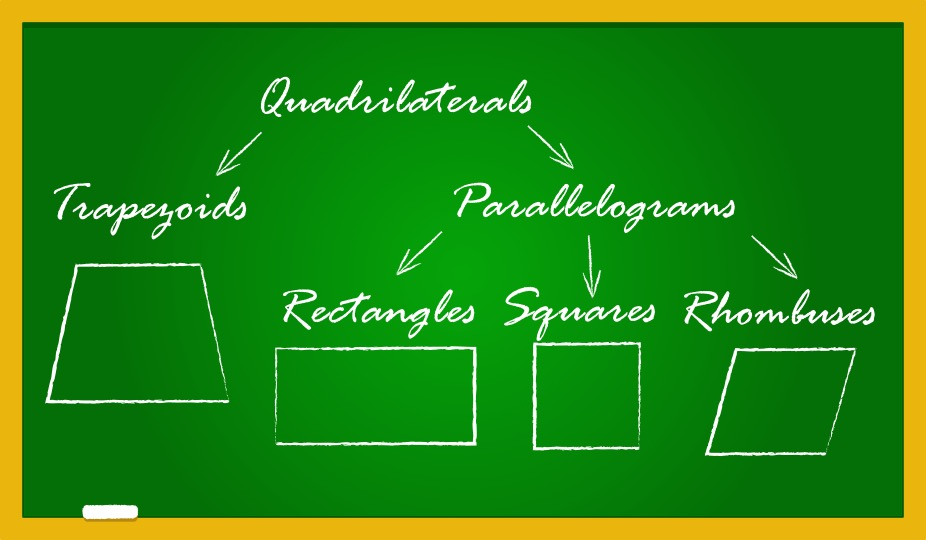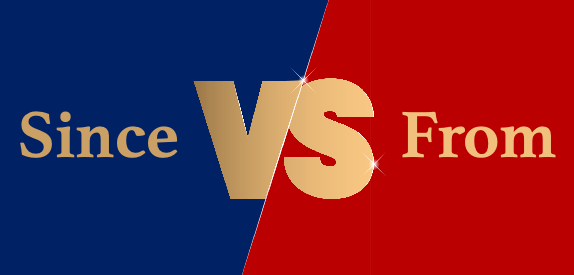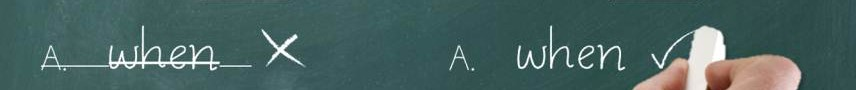# Properties of Triangle – types, formulas, practice problems, video lessons

In this article, we are going to learn about the simplest form of a polygon, a triangle. All polygons can be divided into triangles, or in other words, they are formed by combining two or more triangles. Thus, understanding the basic properties of a triangle and its types is essential. Watch this video to know

Read More# Properties of Quadrilaterals – rectangle, square, parallelogram, rhombus, trapezium

In Euclidean geometry, a quadrilateral is a four-sided 2D figure whose sum of internal angles is 360°. The word quadrilateral is derived from two Latin words ‘quadri’ and ‘latus’ meaning four and side respectively. Therefore, identifying the properties of quadrilaterals is important when trying to distinguish them from other polygons. So, what are the properties

Read More# Lines and Angles – Definitions, Properties, Types, Practice Questions

Ancient mathematicians introduced the concept of lines to represent straight objects which had negligible width and depth. Considered as a breadth less length by Euclid, lines form the basis of Euclidean geometry. When two rays (part of a straight line) intersect each other in the same plane, they form an angle. The point of intersection

Read More# Since vs From – What’s the difference and how to use?

The words since and from both are used to denote the starting time of an action. Yet, these words are used in very different contexts. Use of Since The word since is used to present the starting time of an action that continues in the present till this date. For example: Simple Example 1 Mr.

Read More# GMAT Study Plan – How to study for GMAT 760 efficiently?

Have you ever wondered as to “how to study for GMAT efficiently” or “How can I score a 760 on the GMAT?” Many students ask us for study plans to ace the GMAT. We believe that general study plans are not efficient as every student is different and hence we believe in Personalised Study Plans.

Read More# Should you retake the GMAT? GMAT retake’s why and when in 2021

Michael, Rahul, and Anna – Three people in three different cities took the GMAT on July 1, 2021. All three of them got different scores. While Anna is elated to score a 760, Michael and Rahul are disappointed with their scores of 600 and 650 respectively. They’re both considering retaking the GMAT. While Rahul is

Read More# If Vs. When

In conditional statements, ‘if’ and ‘when’ can be used interchangeably – but only in certain cases.  In certain other cases, using ‘when’ instead of ‘if’ will result in an idiomatically incorrect sentence. In this post, I will explain when ‘if’ and ‘when’ can be used interchangeably and when they cannot be. When in conditional statements can

Read More# The figure above shows a drop-leaf table. With all four leaves down the tabletop is a square, and with all four leaves up the tabletop is a circle. What is the radius, in meters, of the tabletop when all four leaves are up? – OGQR 2020 Question #99 with Solution

OGQR 2020: Question No. 99 The figure above shows a drop-leaf table. With all four leaves down the tabletop is a square, and with all four leaves up the tabletop is a circle. What is the radius, in meters, of the tabletop when all four leaves are up? Source OGQR 2020 Type Problem Solving Topic

Read More# P is a particle on the circle shown above. What is the length of the path travelled by P in one complete revolution around the circle? – OGQR 2020 Question #212 with Solution

Read MoreRead More